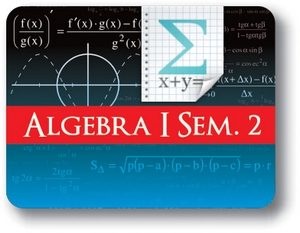# Algebra I Semester 2• Course Credits: 0.5
• Course Price: \$299.00

## Course Overview:

This course allows the student to strengthen their foundation on High School Algebra. Student learns about Functions – their properties, domain, range, identifying functions and evaluating them. The course introduces the student to the System of Linear Equations and teaches them how to solve them. Student learns about Exponents, Radicals and Exponential Functions – properties, interpreting them and simplifying them. They learn about Polynomials and understand various operations with Polynomials including factoring them. The course then delves into Quadratic Equations and Functions. Students explore solving and graphing them. The course provides a solid foundation so that student may proceed confidently to Algebra II.

Note: This course is not designed for ELL (English Language Learners) students. ELL students may enroll in this course ONLY if they have adequate mentor support at their home school and are able to fulfill all course requirements.

## Syllabus:

Section 1: Functions

• Introduction to Functions
• Evaluating and Graphing Functions
• Finding Domain and Range of Functions
• Maximum and Minimum Points
• Positive and Negative Intervals
• Increasing and Decreasing Functions

Section 2: Systems Of Linear Equations

• Introduction to Systems of Equations
• Solving Systems of Linear Equations Using Elimination
• Solving Systems of Linear Equations Using Substitution
• Systems of Inequalities
• Word Problems

Section 3: Exponents, Radicals and Exponential Functions

• Properties of Exponents
• Introduction and Properties of Radicals
• Rational Exponents
• Introduction to Exponential Functions
• Interpreting Exponential Function Formulas and Graphs
• Scientific Notation

Section 4: Polynomials and Factorization

• Introduction to Polynomials
• Operations With Polynomials
• Special Products of Binomials
• Introduction to Polynomial Factorization
• Factoring Monomials and Binomials
• Factor by Grouping

Section 5: Quadratic Equations and Functions

• Solving Quadratics by Taking the Square Root
• Solving Quadratics by Completing the Square

## Awards, Approvals, and Accreditation

••••••••••••••